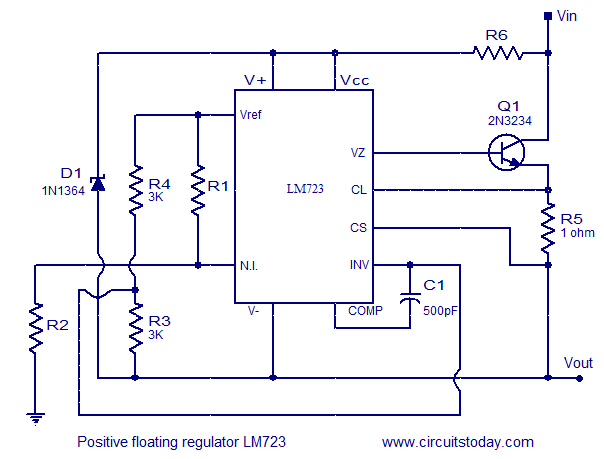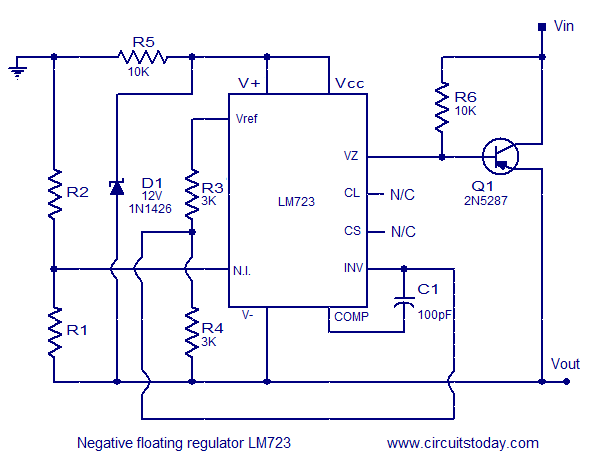## Audio

[Audio][slideshow]

## Floating regulator.

Floating regulator is a type of voltage regulator that works without a real ground connection. This is a trick used for regulating voltages higher than the rated value. For example the typical output voltage range of LM723 voltage regulator IC is 2 to 37V DC. The upper limit can be pushed beyond 37V DC by using the floating technique.

In floating configuration, the ground pin of the regulator will not be at the same voltage of the return path of the load current. This can be achieved by putting a Zener diode in series to the circuit ground. This makes the circuit tricked into working above the real ground to a voltage equal to the breakdown voltage of the Zener diode. The rated safe difference between the unregulated input voltage and regulated output voltage is easily maintained. In simple words, when the regulator is operating in the floating mode, it sees only the difference between the input and output voltages and voltages much higher than the rated value of the regulator can be regulated.

### LM723 floating regulator.

LM 723 is an integrated series regulator from National Semiconductors. Maximum input voltage is 40V and the output voltage range is from 2V to 37VDC. Maximum output current is 150 mA and this can be extended by using external pass transistors. The IC can be configured in linear or switching configuration. The circuit given below is of a positive floating regulator with external pass transistor. Resistor R1 and R2 sets the output voltage. 36V Zener diode D1 is used to trick the circuits ground to operated 36V (break down voltage of D1) above real ground. R5 is the current sensing resistor which is associated with the internal current limiting circuit. Q1 is the series pass transistor. Capacitor C1 is meant for frequency compensation. Output voltage of the circuit is according to the equation: Vout = (Vref/2) x {(R2-R1) /R1}.

#### LM723 positive floating regulator.Positive floating regulator LM723

#### LM723 floating negative regulator.

Circuit diagram of a floating negative regulator is shown below. Output voltage of the circuit is according to the equation: Vout = {Vref/2} x {(R1+R2)/R1}. Working the circuit is almost similar to that of the positive floating regulator. Instead of the NPN pass transistor in former circuit, a PNP pass transistor is used here. A 10K pull up resistor is connected to the base of the pass transistor.Negative floating regulator LM723

[Lights][grids]